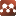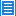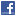## Condensed Matter## Some Problems About The 3He Superfluid

Authors: Ting-Hang Pei

We discuss the stability problem of the atomic pair in the 3He superfluid. It is similar to the bound-state problem of the hydrogen molecule because both of them are composed of two spin-1/2 nuclei. The difference is the cause for bonding. What is of interest here is whether the nuclear spin coupling between two 3He nuclei is large enough to form a stable atomic pair in the superfluid. According to our calculations, the energy difference between the excited state F=1 and the ground state F=0 caused by the nuclear spin coupling of the two hydrogen nuclei is about 5.6x10^-9 eV when the intermolecular distance is 0.74 Å. When the two 3He nuclei are separated by 2.9 Å, the same energy difference caused by the nuclear spin coupling is 4.8x10^-10 eV. This value is much less than the binding energy of two 3He atoms about 1.0 eV, and is also much smaller than the Lennard-Jones potential of 8.74x10^-4 eV between the two helium atoms. Therefore, two 3He atoms cannot form a stable atomic pair due to the nuclear spin coupling even the spin wave.